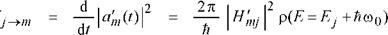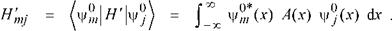## Quantum mechanical model of recombinationW

Spontaneous recombination based on quantum mechanics was discussed by Bebb and Williams (1972), Agrawal and Dutta (1986), Dutta (1993), Thompson (1980), and others. The quantum mechanical calculation for the spontaneous emission rate is based on the induced emission rate given by Fermi’s Golden Rule which gives the transition probability per unit time (called transition rate) from a quantum mechanical state j to a state m

(3.1)where Hmj' is the transition matrix element. For the one-dimensional case with a dependence on only the spatial variable x, the matrix element that connects the (initial) jth state with the (final) mth state via the perturbation hamiltonian H', is given by

(3.2)

For the derivation of Fermi’s Golden Rule, the perturbation hamiltonian H' is assumed to have a harmonic time dependence, i. e. H' = A(x) [ exp (io0t) + exp (-ira0t) ], as expected for the excitation by a harmonic wave of a photon. Equation (3.2) indicates that a necessary condition for recombination is spatial overlap between electron and hole wave functions. This is intuitively

clear, because spatially separated electrons and holes will not be able to recombine.

For optical transitions between the conduction and valence band, the electron momentum must be conserved because the photon momentum (p = hk) is negligibly small. The conservation of momentum condition is known as the k-selection rule. For the remainder of this section, we closely follow the quantum mechanical analysis given by Agrawal and Dutta (1986) and the reader is referred to that reference for a comprehensive discussion.

The average matrix element for the Bloch states, | Mb | can be derived using the four-band Kane model (Kane, 1957), which takes into account the conduction, heavy-hole, light-hole, and split-off band. In bulk semiconductors | Mb |2 is given by (Kane, 1957; Casey and Panish, 1978)

 Mb2
 (3.3)

me2 Eg (Eg + A)

12me (Eg + 2A/3)

where me is the free-electron mass, Eg is the band gap, and A is the spin-orbit splitting. For GaAs, using Eg = 1.424 eV, A = 0.33 eV, me* = 0.067 me, we get | Mb |2 = 1.3 me Eg.

Taking into account the k-selection rule, the total spontaneous emission rate per unit volume is given by

 2

 V

 rsp (E) =

 (2 п)3

 V

 2 4пne E 2 2 3 me Sq h c

(3.4)

 X

^ Jfc(Ec) fv(Ev) d3kc d3kv 5(kc - ку) 5(Ei - Ef - E)

where fc and fv are the Fermi factors for electrons and holes, and the term 5 (kc - kv) ensures satisfaction of the k-selection rule. The factor 2 arises from the two spin states. In Eq. (3.4), £ stands for the sum over the three valence bands (heavy-hole, light-hole, and split-off bands). For definiteness, we first consider transitions involving electrons and heavy holes. The integrals in Eq. (3.4) can be evaluated with the following result:

 2ne EMb| 2 2 3 п me Sq h c

 rsp (E) =

 (3.5)

 2 3/2 -m-l JE - Eg fc( Ec) fv (Ev) h j

where

Ec = (mr/m* ) (E - Eg) , (3.6)

 Ev = mr

(mr/ m*h )(e - Eg ) , (3 7)

mr = me mhh* , (3.8)

me + mhh

and mhh* is the effective mass of the heavy hole. Equation (3.5) gives the spontaneous emission rate at the photon energy E. To obtain the total spontaneous emission rate, a final integral should be carried out over all possible energies. Thus the total spontaneous emission rate per unit volume due to electron-heavy-hole transitions is given by

R = 1Г rsp(E) dE = A |Mb I2 I (3.9)

Eg

where

Г Г і-----------

I = 1 Eg l/E - Eg fc(Ec) fv(Ev)dE (3.10)

and A represents the remaining constants in Eq. (3.5). A similar equation holds for the electron-light-hole transitions if we replace mhh* by the effective light-hole mass mlh*.

The quantum mechanical absorption coefficient a(E) derived by Agrawal and Dutta (1986) using a similar analysis is given by

e2hMb|2 ( 2m, ''n I--------- r,

a(E) = 2 f2b^------------- f - JE - Eg [1 - fc(Ec) - fv(Ev) ]. (3.11)

 v h2 J

22

4n Som^cnE

Although the quantum mechanical model of recombination is most appropriate and accurate, it can be time-consuming and awkward to deal with. The following sections analyze recombination in semi-classical terms that usually are more convenient to work with.

Комментарии закрыты.